# Reciprocity Theory

It is the relationship between total cross section σ (x-> y) of the entrance channel x and total cross section σ (y->x) of reaction channel y.

Reciprocity theory is based on the idea that the probability of a reaction occurring is proportional to the flux of particles incident on the target nucleus. By measuring the flux of particles incident on the target and the number of particles scattered or absorbed by the target, the cross section for the reaction can be determined. Reciprocity theory allows this measurement to be related to the cross section for the same reaction in the opposite direction.

Reciprocity theory is an important tool for experimental nuclear physicists, as it provides a way to check the consistency of their measurements and to compare data from different experiments. It is also used in theoretical nuclear physics to develop models of nuclear reactions and to predict cross sections for new reactions. Let us consider a reversible process

`X +x ⇌ Y + y ........1`

in which X, x, Y, y all occur in arbitrary number in a large box of volume V.

The number of channels is given momentum range dp in the box of volume V in the equilibrium. condition is given by

N = 4πVP²dP/ ℏ³ = VP²dP / 2π²ℏ³

Since E = P²/ 2m

or, 2m dE = 2P dP

or, dP = mdE /P = mdE/ mv = dE/v

Therefore, the number of channels in the box of volume V in the range dE in equilibrium condition is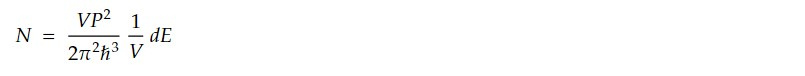Here, we have two channels; x and y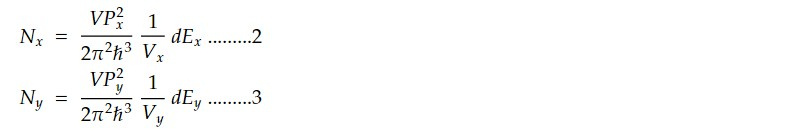The energy range for the two channels must be same i.e dEx = dEy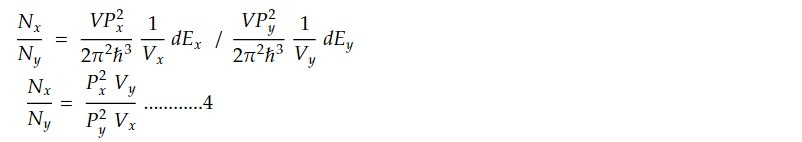The number of transitions x-> y per sec = N_x w (x -> y). Similarly, the number of transitions y-> x per sec = N_yw (y -> x) are the transition probabilities for the reactions (x-> y) and (y->x) respectively.

The system is said to be dynamical equilibrium when the number of transitions x->y per sec is equal to number of transitions y->x per sec and is known as detailed balance. Thus in dynamical equilibrium condition, we have,

N_x w(x-> y) = N_yw (y -> x) ......5

Since the transition probability measures the chance that particle moving with velocity 'v ' in a volume V is scattered per sec, cross section σ which corresponds to unit flux is

σ = wV/ v

=> w = σ v/ V ....6

From equation 5 and 6,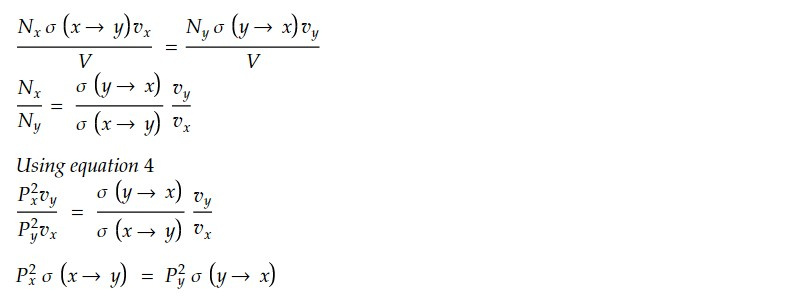Using P = ℏk we get

`kx² σ (x -> y) = ky² σ (y -> x).......7`

If there are intrinsic angular momentum (I) for x, X, y, Y we may write equation 7 as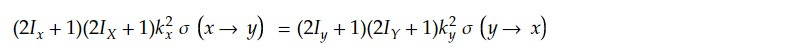Here, intrinsic angular momentum, or spin, plays an important role in reciprocity theorem in nuclear physics. The theorem takes into account the effects of intrinsic spin when relating the response of a detector to incident radiation with the response of the same detector when it is used as a source of radiation.

This note is a part of the Physics Repository.